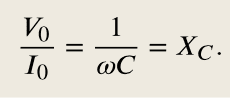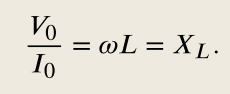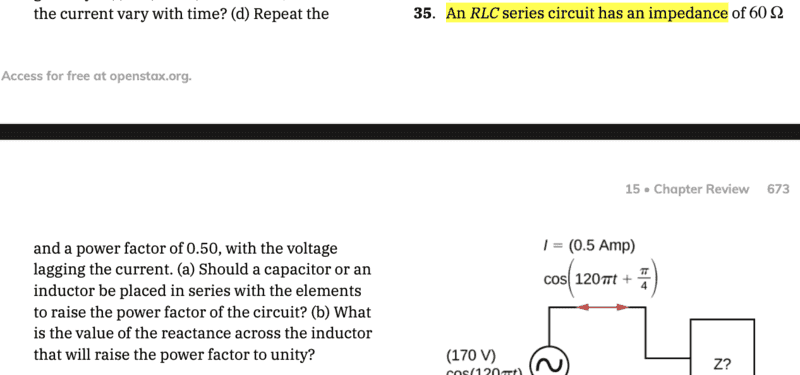# Adjusting the Power Factor of an RLC circuit

annamal
Homework Statement:
An RLC series circuit has an impedance of 60 ohms and a power factor of 0.50, with the voltage lagging the current. (a) Should a capacitor or an inductor be placed in series with the elements to raise the power factor of the circuit? (b) What is the value of the reactance across the inductor that will raise the power factor to unity?
Relevant Equations:
##P = 0.5\times IVcos(\phi)##

##Z = \sqrt{R^2 + (X_l - X_c)^2}##
a) An inductor should be added because that would cancel out the lag of the voltage with the current so that P = IV is at a maximum since V is ahead of I in an inductor
b) ##cos(\phi) = \frac{R}{Z}##, ##R = Zcos(\phi) = 60\times 0.5 = 30 ohms##
##X_l - X_c = \sqrt{Z^2 - R^2} = \sqrt{60^2 - 30^2}## = 52 ohms... don't know how to solve any longer

alan123hk
Unity power factor means ##cos(\phi) ## needs to be equal to 1, so there is no lag or lead b/w voltage and current. 🤔

Gold Member
Are you familiar with representing impedance with complex numbers, like ##Z_L = j \omega L## , or ##Z_C = \frac{1}{j \omega C}## ?

annamal
Unity power factor means ##cos(\phi) ## needs to be equal to 1, so there is no lag or lead b/w voltage and current. 🤔
Yeah I get that. So R = Z, but I still don't know how to solve for ##X_L##

annamal
Are you familiar with representing impedance with complex numbers, like ##Z_L = j \omega L## , or ##Z_C = \frac{1}{j \omega C}## ?
Yes, I am familiar with that, without the j that is.

alan123hk
Yeah I get that. So R = Z, but I still don't know how to solve for ##X_L##

Now you know that you need to add a new inductor or replace the current one with a larger inductance, you also know that before adding the new inductor, the reactance difference between the original capacitor and the inductor is 52 ohms, I'm a little surprised, you still want to know What?

Is it the reactance of the newly added inductor or the total reactance of the newly added inductor in series with the original inductor?Mentor
Yes, I am familiar with that, without the j that is.
Wait, what? That makes no sense. And those equations make no sense without the "j" complex number qualifer.

Gold Member
Yes, I am familiar with that, without the j that is.
So then, no. The ##j## is the whole point of complex numbers. ##j = \sqrt{-1}##, many people also use ##i = \sqrt{-1}## instead, it's the same thing. You WILL end up learning this at some point fairly soon, I think. It's the easy way to deal with circuit impedance.

That's OK, we just need to know to explain this the best way.

So, if the total (net) impedance seen by the source is inductive then the voltage leads the current. If it's capacitive the voltage lags the current. But for unity power factor the voltage should neither lead or lag the current, but be in phase. So given that the reactive impedance of a capacitor can cancel (or subtract from) the reactive impedance of an inductor (and vice-versa). What is the amount of additional reactance required to make the inductive reactance cancel the capacitive reactance?

Gold Member
While you may not be familiar with complex numbers the reactance (impedance) of the capacitor works like this:
##Z_C = \frac{1}{j \omega C} = \frac{1}{\sqrt{-1} \omega C} = ( \frac{\sqrt{-1}}{\sqrt{-1}})( \frac{1}{\sqrt{-1} \omega C}) = - \sqrt{-1} \frac{1}{\omega C} = -j \frac{1}{ \omega C}##

Then you want ##|Z_C| = |Z_L| \Rightarrow \frac{1}{ \omega C} = \omega L## so that when you add them up ##Z_C + Z_L = -j \frac{1}{ \omega C} + j \omega L = j(- \frac{1}{ \omega C} + \omega L) = 0##

But then, of course, the total impedance of the series RLC is ##Z = R + Z_C + Z_L##

annamal
Wait, what? That makes no sense. And those equations make no sense without the "j" complex number qualifer.
My book defines them here:
https://openstax.org/books/university-physics-volume-2/pages/15-2-simple-ac-circuits as equations 15.3 and 15.8annamal
While you may not be familiar with complex numbers the reactance (impedance) of the capacitor works like this:
##Z_C = \frac{1}{j \omega C} = \frac{1}{\sqrt{-1} \omega C} = ( \frac{\sqrt{-1}}{\sqrt{-1}})( \frac{1}{\sqrt{-1} \omega C}) = - \sqrt{-1} \frac{1}{\omega C} = -j \frac{1}{ \omega C}##

Then you want ##|Z_C| = |Z_L| \Rightarrow \frac{1}{ \omega C} = \omega L## so that when you add them up ##Z_C + Z_L = -j \frac{1}{ \omega C} + j \omega L = j(- \frac{1}{ \omega C} + \omega L) = 0##
Yes, and right now the ##Z_L - Z_C= 52##, so the new ##Z_L## has to be -52?

Gold Member
Your book has buried the sign of the reactance into the words leading/lagging or something similar. They (or you) have left out the magnitude operator. What they should have said (or maybe did) was ## |\frac{V_0}{I_0}| = \frac{1}{\omega C} = X_C = |Z_C| ##.

PS: OK, plus, sorry to be snotty, but nobody should call a physics book "University Physics" and teach this subject without complex numbers. They are making it unnecessarily difficult by not waiting until you have the math background to make it easy to understand. This is not how this stuff is taught in the universities I am familiar with. JMHO.

Last edited:
•berkeman
Gold Member
Yes, and right now the ##Z_L - Z_C= 52##, so the new ##Z_L## has to be -52?
Sorry, I haven't actually looked at the numbers. But...
You are being sloppy with your definitions. I don't understand "new ##Z_L##", give them different names or at least describe them with words. If it's a different inductor, it needs a different name. Do you mean you are adding an inductor with ##|Z_{L'}| = X_{L'} =-52##? How does that work? How do you get a negative value for ##|Z_{L'}| = \omega L' ##?

annamal
Sorry, I haven't actually looked at the numbers. But...
You are being sloppy with your definitions. I don't understand "new ##Z_L##", give them different names or at least describe them with words. If it's a different inductor, it needs a different name. Do you mean you are adding an inductor with ##|Z_{L'}| = X_{L'} =-52##? How does that work? How do you get a negative value for ##|Z_{L'}| = \omega L' ##?
Yes, I am adding an inductor with ##|Z_{L'}| = X_{L'} = -52## so that ##X_L - X_C## = 0

Gold Member
OK, I'll give you a break on the whole sign thing. Except to point out that magnitudes are never negative and inductance is never negative. Reactance can be negative, but that would be a capacitor with a non-negative capacitance. This is an important issue for you to get straight someday, but will be easier when you are comfortable with complex impedance values.

So, yes, adding a series inductor with a reactance X = +52, or and inductance of L = 52/ω will give you unity power factor.

But... Now that I've finally read the question carefully, I wonder if you copied it correctly? Part b) asks "What is the value of the reactance across the inductor that will raise the power factor to unity?". Compensating the reactance by adding a component "across the inductor" isn't a solvable problem without knowing the value of the original L or C, we only know the net reactance, the difference between the inductive and capacitive parts.

At best it's a poorly worded question. All we can know is that we have to add, not what the original individual reactive values were.

•Delta2
alan123hk
••DaveE and Delta2
Staff Emeritus
Homework Helper
Gold Member
Homework Statement:: An RLC series circuit has an impedance of 60 ohms and a power factor of 0.50, with the voltage lagging the current. (a) Should a capacitor or an inductor be placed in series with the elements to raise the power factor of the circuit? (b) What is the value of the reactance across the inductor that will raise the power factor to unity?
Relevant Equations:: ##P = 0.5\times IVcos(\phi)##

##Z = \sqrt{R^2 + (X_l - X_c)^2}##

a) An inductor should be added because that would cancel out the lag of the voltage with the current so that P = IV is at a maximum since V is ahead of I in an inductor
b) ##cos(\phi) = \frac{R}{Z}##, ##R = Zcos(\phi) = 60\times 0.5 = 30 ohms##
##X_l - X_c = \sqrt{Z^2 - R^2} = \sqrt{60^2 - 30^2}## = 52 ohms... don't know how to solve any longer
Be careful in solving this equation, ##~~\displaystyle Z = \sqrt{R^2 + (X_L - X_C)^2} ## ,

especially when solving for ##X_L-X_C## .

Squaring both sides gives : ##~~\displaystyle Z^2 = R^2 + (X_L - X_C)^2 ## .

Solving for ##(X_L-X_C)^2 ## gives: ##~~\displaystyle (X_L - X_C)^2 = Z^2 - R^2 ## .

When taking the square root of both sides, you must remember to supply a ± sign.

So you get ##~~\displaystyle X_L - X_C = \pm\sqrt{Z^2 - R^2~} ## .

The sign is determined by knowledge of what is lagging or leading.

Voltage versus Current

•annamal and Delta2
annamal
OK, I'll give you a break on the whole sign thing. Except to point out that magnitudes are never negative and inductance is never negative. Reactance can be negative, but that would be a capacitor with a non-negative capacitance. This is an important issue for you to get straight someday, but will be easier when you are comfortable with complex impedance values.

So, yes, adding a series inductor with a reactance X = +52, or and inductance of L = 52/ω will give you unity power factor.

But... Now that I've finally read the question carefully, I wonder if you copied it correctly? Part b) asks "What is the value of the reactance across the inductor that will raise the power factor to unity?". Compensating the reactance by adding a component "across the inductor" isn't a solvable problem without knowing the value of the original L or C, we only know the net reactance, the difference between the inductive and capacitive parts.

At best it's a poorly worded question. All we can know is that we have to add, not what the original individual reactive values were.
This is the problem exactly (#35):annamal
Be careful in solving this equation, ##~~\displaystyle Z = \sqrt{R^2 + (X_L - X_C)^2} ## ,

especially when solving for ##X_L-X_C## .

Squaring both sides gives : ##~~\displaystyle Z^2 = R^2 + (X_L - X_C)^2 ## .

Solving for ##(X_L-X_C)^2 ## gives: ##~~\displaystyle (X_L - X_C)^2 = Z^2 - R^2 ## .

When taking the square root of both sides, you must remember to supply a ± sign.

So you get ##~~\displaystyle X_L - X_C = \pm\sqrt{Z^2 - R^2~} ## .

The sign is determined by knowledge of what is lagging or leading.

Voltage versus Current
I see, since voltage is lagging current, there is more of capacitor voltage and less of inductor voltage, leading to the negative sign of ##X_L - X_C##, so you have to add ##X_L## of +52 ohms

•DaveE
Staff Emeritus
•